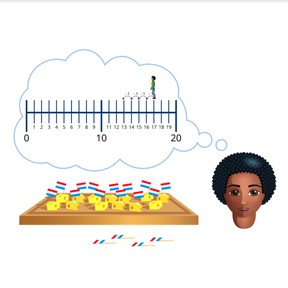Subtraction to 20 on the number line

# Subtraction to 20 on the number line

No account needed.8,000 schools use Gynzy92,000 teachers use Gynzy1,600,000 students use Gynzy

## General

Students learn to subtract numbers to 20 by making use of a number line.

1.OA.C

## Relevance

It is important to be able to subtract to be able to determine how many you have left after you take a quantity away.

## Introduction

Count from one to twenty, but make a mistake somewhere in your counting by skipping a number. Tell students to clap when they hear a mistake. To challenge your class, tell them to close their eyes and raise a hand when they hear a mistake in your counting.

## Development

Start by discussing how to subtract using the fish on the interactive whiteboard. Then using three steps, explain how to subtract using a number line. First students must look at the first number (minuend) of the subtraction problem and move to that number on the number line. Secondly, students must look at the second number (subtrahend) of the subtraction problem to determine how many steps they must jump back on the number line. The third step is to determine which number they end at, which is their difference. To check that students can subtract using the number line, ask students to complete the subtraction problems given on the interactive whiteboard as a class. Remind students that they can choose how big to make their jumps back on the number line (by one, two, etc).

To check that students are able to subtract numbers to 20 using the number line, you can ask the following questions:
- Which number in a subtraction problem do you look at to determine where you start on the number line?
- Which number in a subtraction problem do you look at to determine how many jumps back you take?
- How do you know what the difference is?

## Guided practice

Students practice subtraction using the number line, and the numbers start smaller and gradually increase.

## Closing

Remind students why it is important to be able to subtract numbers to 20. Next the interactive whiteboard shows a subtraction problem and a number line. Students must determine what the difference is by deciding how many jumps they take. Ask students to discuss in pairs/groups how they determined the difference and then discuss as a class.

## Teaching tips

If students have difficulties with this goal, it is important to help them by supporting their knowledge of the number line to 20. Ask them to draw the number line to 20 and to write all the numbers from 0 to 20 beneath each mark on the number line. Then have students practice by taking one jump back from a given number to gain comfort and familiarity with the process.

### The online teaching platform for interactive whiteboards and displays in schools

• Save time building lessons

• Manage the classroom more efficiently

• Increase student engagement